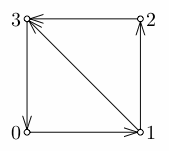### Problem Statement

There are several cities in a country, and some pairs of those cities are connected by one-way roads (although it is possible that there are one-way roads both from A to B and from B to A). It is possible to get from any city to any other city using roads. A traveler starts from some city, and travels along one of the roads every day. A number x is called vacation-friendly if a traveler can have an x-day long vacation. That means he can start from some city, travel exactly x roads, and be back at the city where he started.

A vacation string is an infinite string of '0' and '1' digits, which has a '1' in the x-th (1-based) position if and only if x is a vacation-friendly number.

For example, consider the country shown in the following picture.Its vacation string is "0011011111111...": 0->1->3->0 is a 3-day vacation, 0->1->2->3->0 is a 4-day vacation, 0->1->3->0->1->3->0 is a 6-day vacation, 0->1->2->3->0->1->3->0 is a 7-day vacation, and so on.

It turns out that every vacation string becomes periodic at some point. We will enclose the period in parentheses to obtain a finite representation for a vacation string. For example, the above vacation string can be represented by "00110(1)" or "00110111(11)". We will call the shortest possible representation canonical.

Given a description of a country as a String[] arcs, where the j-th character of the i-th element of arcs is 'Y' if there is a road from city i to city j, and 'N' otherwise, return the canonical representation of its vacation string.

### Definition

 Class: AllCycleLengths Method: findAll Parameters: String[] Returns: String Method signature: String findAll(String[] arcs) (be sure your method is public)

### Constraints

-arcs will contain between 2 and 30 elements, inclusive.
-Each element of arcs will contain exactly n characters, where n is the number of elements in arcs.
-Each character of each element of arcs will be either 'Y' or 'N'.
-The i-th character of the i-th element of arcs will always be 'N'.
-arcs will define a network of roads where it is possible to get from any city to any other city using the roads.

### Examples

0)

 `{"NYNN", "NNYY", "NNNY", "YNNN"}`
`Returns: "00110(1)"`
 The example from the problem statement.
1)

 `{"NY", "YN"}`
`Returns: "(01)"`
 Only even numbers are vacation-friendly here.
2)

 `{"NYYYY", "NNYYY", "NNNYY", "NNNNY", "YNNNN"}`
`Returns: "0(1)"`
 Every vacation length except 1 is possible here.
3)

 `{"NYNNN", "NNYNN", "NNNYN", "NNNNY", "YNNYN"}`
`Returns: "010(1)"`
 The vacation can start from any vertex.

#### Problem url:

http://www.topcoder.com/stat?c=problem_statement&pm=9764

#### Problem stats url:

http://www.topcoder.com/tc?module=ProblemDetail&rd=12177&pm=9764

Petr

#### Testers:

PabloGilberto , Olexiy , Jan_Kuipers , ivan_metelsky

#### Problem categories:

Graph Theory, String Manipulation